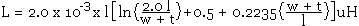# Flat Wire Inductor Calculator

The inductance of a flat or ribbon wire (rectangular cross section), can be found using the calculator or the equation given below.

 INPUT DATA Length: cm Width: cm Thickness: mm
 RESULTS Inductance: nH

This calculator uses JavaScript and will function in most modern browsers. For more information see About our calculators

The inductance of a flat or ribbon wire or a track on a PCB can be calculated with the following equation.Where l is the Length in cm

w is the Width in cm

t is the thickness in cm (PCB copper @ 1 oz/ft2 = 0.035mm)

The original for this equation is by F.E. Terman and can be found in the Radio Engineers Handbook, McGraw-Hill, New York, 1945. The units in the calculator have been changed from µH to nH and the thickness from cm to mm for easier working on PCB's.

This calculator is provided free by Chemandy Electronics in order to promote the FLEXI-BOX